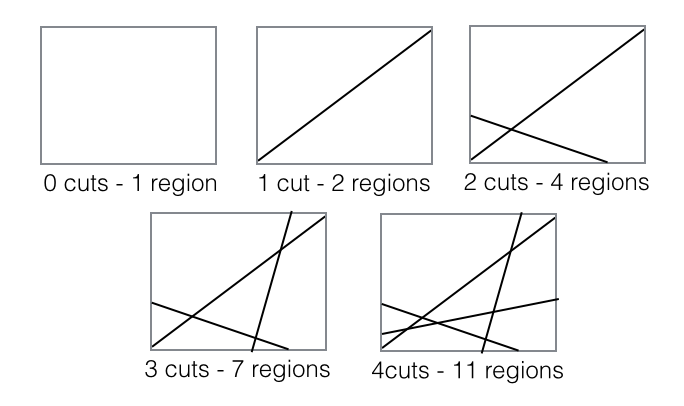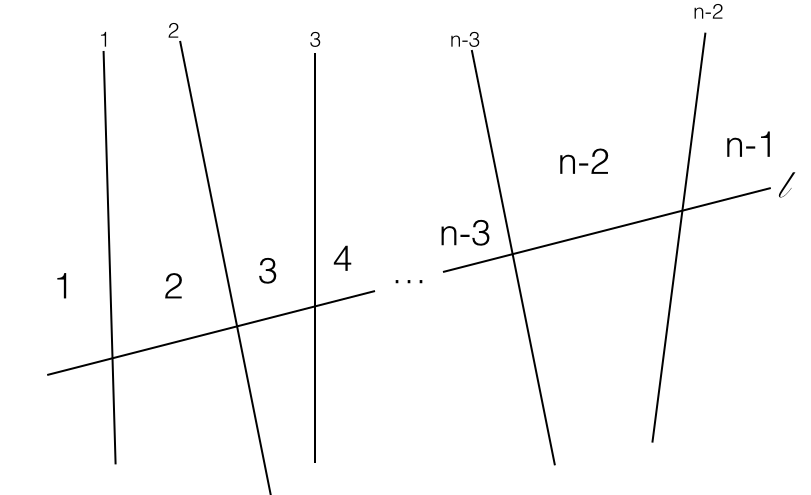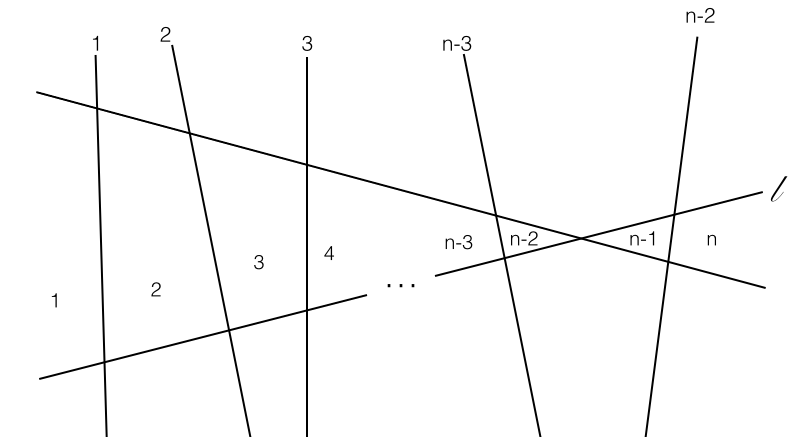## Slicing a Plane

2017 Feb 19

What is the largest number of pieces into which you could slice a plane with $n$ line cuts?

Suppose we are tasked with finding a general rule for the maximum number of regions that a plane can be sliced into with $n$ line cuts. First we need to decide what a "perfect" series of slices looks like, or the series of slices producing the largest number of regions. First, in order to create as many new regions as possible, each line drawn should intersect each other line. Additionally, no two lines should intersect another line at the same point (since more regions could be obtained if they intersect in different locations).

First, we observe the first couple values, and then we may begin to attack the problem.Suppose a plance has already been sliced $n-1$ times so that it has been divided into the maximum number of regions that can be made with $n-1$ cuts, or so that each line intersects each other line and no three lines intersect at the same point. Choose one of the lines $l$ that has already been constructed. Then there are $n-2$ lines intersecting $l$ each at different points. We can see from the picture that $l$ is touching $n-1$ of the regions already made on each of its sides:A new line can be constructed by finding a rotation of $l$ at a point at which it does not intersect any other lines so that it is not parallel to any preexisting lines, and so that it does not pass through any other points of intersection. Such a line intersects each other line at distinct points, so it adds the maximum number of possible regions, which we can see from the visual is a total of $n$ new regions.If $r_n$ denotes the maximum number of regions after slice $n$, we have just established the property

Since we also know that

$r_0=1$

We have a recursive definition of $r_n$, which we can use to find an explicit one. We already have that

which can be rewritten as

Since the formula for the sum of the first $n$ integers is $\frac{n(n+1)}{2}$, we can replace that with

Which is the formula for the maximum number of regions after $n$ slices.